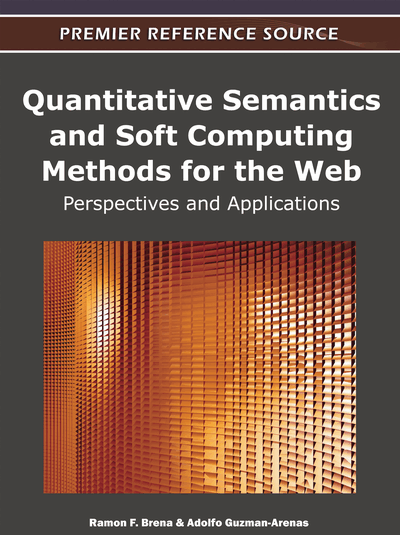# Clustering Via Centroids a Bag of Qualitative values and Measuring its Inconsistency

Adolfo Guzman-Arenas (Instituto Politécnico Nacional, México) and Alma-Delia Cuevas (Instituto Politécnico Nacional, México)
DOI: 10.4018/978-1-60960-881-1.ch001
Available
\$37.50
No Current Special Offers

## Abstract

All observers are equally credible, so differences in their findings arise from perception errors.
Chapter Preview
Top

## 1. Previous Work And Problem Statement

Our work is in the general area of extracting useful properties (such as “centers” and “clusters”) from a set of non-numeric values.

### 1.1 Problem Statement

Assume several measurements are performed on the same property (for instance, the length of a table). One measurer took a quick look and asserted “3m.” Another person with the help of a meter said “3.13m”. A lady with a micrometer reported “3.1427m.” The problem of finding the average of a set of quantitative values (to be called “Problem 0”) can be solved simply by computing the average (μ=3.09m, the average length) as well as the dispersion of these measurements (σ, the variance), perhaps disregarding some outliers. For quantitative measurements we know how to take into account contradicting facts, and we do not regard them necessarily as inconsistent. We just assume that the observers’ gauges have different precisions or accuracies.

It could also be that observers have a propensity to lie, and in this case we apply the Theory of Evidence (Dempster, A. (1968); Shafer, G. (1976)). Or we could use Fuzzy Logic, selecting some sets as possible answers and assigning a degree of membership to each measurement for each set.

Problem 1 statement (informal). Similar to Problem 0, we want to solve the problem of finding the “average,” most plausible value, or centroid of several non-numeric or symbolic values.1 This is “Problem 1,” solved elsewhere (Guzman-Arenas, A., & Jimenez, A. (2010)) and briefly exposed in §1.4. There we find that the centroid is the value that minimizes the total confusion in the bag, a number that tells us how “comfortable” the elements of the bag with the chosen centroid are. Nevertheless, according to the problem at hand, it may be possible to have more than one “average.” A bag, thus, may have several centroids, each of them representing or being “the center” of a cluster.2 Several symbolic values in a bag could be better represented (in the sense of a smaller total confusion for the bag) by more than one centroid. Thus, we would like to cluster a bag of values into several centroids. This is “Problem 2” and its formal statement and solution (in Section 2) is the subject of this chapter.

## Complete Chapter List

Search this Book:
Reset